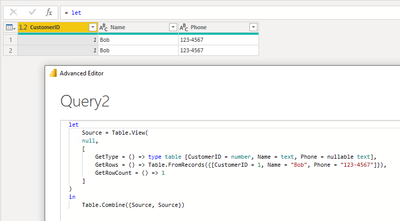cancel
Showing results for
Did you mean:Helper II

## Table.Combine Dies With Stack Overflow

Hello Microsoft Team,

I've stumbled across a stack overflow error which rears its ugly head if I try to Table.Combine two tables which are both based on Table.View. 😞

Any idea what's going on here, or suggestions on how we can prevent this? Is this a Power Query bug?

Cutting it down to the relevant code, here's a repo example which can be demoed in Power BI's Query Editor:

``````let
GetView = (state as record) =>
Table.View(
null,
[
GetType = () => Value.Type(GetRows()),
GetRows = () => #table({"Col1"}, {{"a"}}),
OnTake = (count as number) => @GetView(state & [Take = count])
]
)
,
Table = GetView([])
in
// * The following successfully return a table:
// Table
// Table.FirstN(Table, 1000)

// * All of the following die with: 'Expression.Error: Evaluation resulted in a stack overflow and cannot continue.'
// Table.FirstN(Table.Combine({Table, Table}), 1000)
// Table.Combine({Table.FirstN(Table, 1000), Table.FirstN(Table, 1000)})
Table.Combine({Table, Table})``````

The self-reference (i.e. the above combining Table with itself) doesn't appear to be the issue, as the stack overflow also occurs when two different views are involved:

``````let
GetViewA = (state as record) =>
Table.View(
null,
[
GetType = () => Value.Type(GetRows()),
GetRows = () => #table({"Col1"}, {{"a"}}),
OnTake = (count as number) => @GetViewA(state & [Take = count])
]
)
,
GetViewB = (state as record) =>
Table.View(
null,
[
GetType = () => Value.Type(GetRows()),
GetRows = () => #table({"Col1"}, {{"a"}}),
OnTake = (count as number) => @GetViewB(state & [Take = count])
]
)
in
Table.Combine({GetViewA([]), GetViewB([])})``````

Help here would be much appreciated! Thank you!

4 REPLIES 4Community Support

Hi @Ben-Dev

I tested with a simple Table view. It can be combined with itself.Perhaps the issue is due to the code for generating your table view?

Best Regards,
Community Support Team _ JingHelper II

Thanks, @v-jingzhang!

I believe the issue here may be related to the combination of Table.Combine and OnTake. From what I'm hearing, sounds like there's an underlying issue which I'll just have to live with.Super User

I don't know if this helps whatever your ultimate goal is but you can avoid the error by buffering Table to memory.

That is, replace

``Table = GetView([])``

with

``Table = Table.Buffer(GetView([]))``Helper II

Hi @AlexisOlson, thanks!

Unfortunatley, since buffering blocks subsiquent folding and the real-life Table.View handles more complex folding than is shown in the example, I'm not sure I can realistically use buffering.Announcements#### Exclusive opportunity for Women!

Join us for a free, hands-on Microsoft workshop led by women trainers for women where you will learn how to build a Dashboard in a Day!#### Power Platform Conference-Power BI and Fabric Sessions

Join us Oct 1 - 6 in Las Vegas for the Microsoft Power Platform Conference.Top Solution Authors
Top Kudoed Authors
Users online (2,896)